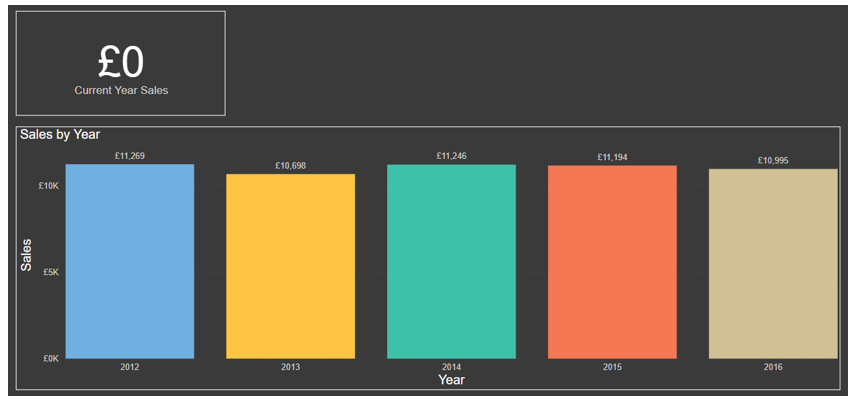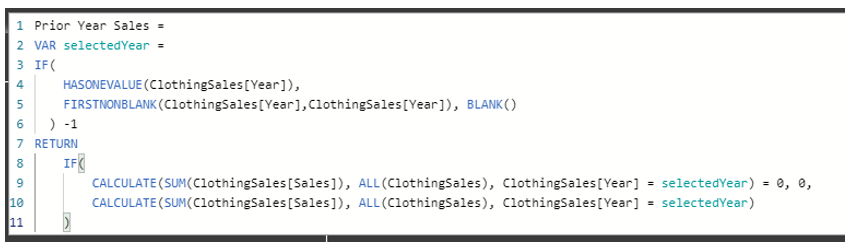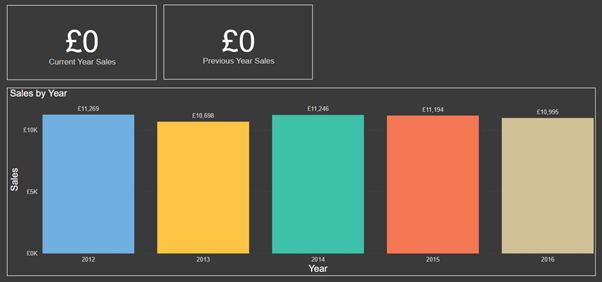cancel
Showing results for
Did you mean:## Current & Previous Year Sales Based on a Selection in Power BI

A workaround to fulfil the requirement of displaying the current year sales and previous year sales, based on a selection of a single data point on year value in a bar chart visual. Let us see how we can achieve this quickly in Power BI.

Let us consider the following bar chart visual, which visualizes the total sales over years.Firstly, let us create a measure for the selected year’s sales.In the above measure, I am taking summation of my sales column, within an IF condition. IF condition here checks if a value is selected on YEAR column, then display the total sales value for that year, otherwise display 0.

Let us create a card visual and move this measure to it. I have labelled this card as “Current Year Sales”.Now, whenever I select a year bar from the bar chart, the card visual shows the selected year’s total sales value.Now let us move to the Previous Year sales measure calculation.1. 1st Line is the name of the measure.
2. 2nd to 6th Line – Here we are creating a variable to get the previous year from the selected year. HASONEVALUE checks if a value is selected and returns that value; then subtracts 1 from the returned value. Basically, if 2013 is selected, then 2013 – 1 is returned, i.e. 2012.
3. 7th Line is the declaration for RETURN statement.
4. 8th to 11th Line – On line 9th we check a condition if the selected year is non-zero value or not. If it is ZERO, then total sales returned is 0. The else condition of IF statement returns total sales for the non-zero selected year.

After moving this to a card visual, we get the following:Now, let us see how this is working, when we select a year bar on the bar-chart.In the above short video, when I select 2013, the Current Year Sales display the 2013 year’s total sales value & Previous Year Sales display the 2012 year’s total sales value.

Just to add a little bit to the user experience I added the following card: (Select Year to View Current & Previous Year Sales)A simple measure was added to get this:Let us see the complete functionality in a single frame:This is how we can get current year sales and previous year sales based on a selection from the visual. This approach can be implemented using other charts as well like Pie chart, Line chart, Table visual, etc.